# Find determinant of a matrix in C++

In this tutorial, we will learn how to find the determinant of a matrix in C++.

## Determinant of a Matrix

Determinant of a Matrix: is a special number that can be calculated from elements of a square matrix ( a matrix having equal no. of rows and columns).

The determinant of a square matrix A is denoted by det A or | A |.

Things to keep in mind:

1.  Determinant only exists for a square matrix.
2.  The determinant can be a negative number.

For a 1 x 1  Matrix

For a  1 x 1  matrix ( 1 row and 1 column )=>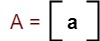The determinant is |A| = a.

For a 2 x 2  Matrix

For a  2 x 2  matrix ( 2 rows and 2 columns )=>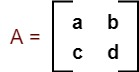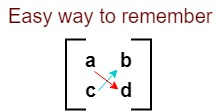The determinant is |A| = ad – bc.

For a 3 x 3 Matrix

For a  3 x 3  matrix ( 3 rows and 3 columns )=>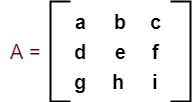The determinant is |A| = a( ei – fh ) – b( di – gf ) + c( dh – eg ).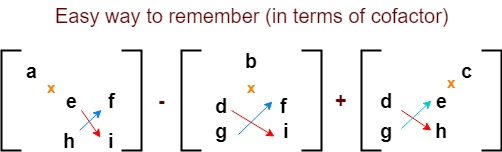Cofactor of an element: is a number associated with an element in a square matrix, equal to the determinant of the matrix formed by removing the row and column in which the element appears from the given determinant.

## Program to find determinant of a matrix in C++

```#include<iostream>
#include<math.h>       // used for pow() function. pow() function is used to calculate some power of a number
using namespace std;

int find_determinant(int matrix, int n)
{
int a,b,c,d,i,j,k;
int temp_determinant=0,subcofactor_matrix,cofactor_i,cofactor_j;

if(n==1)          // if order of a matrix is 1
return matrix;

if(n==2)         // if order of a matrix is 2
{
a=matrix;
b=matrix;
c=matrix;
d=matrix;

return (a*d - b*c);
}

if(n==3)        // if order of a matrix is 3
{
// loop for 0th row elements. Because for finding determinant of a matrix we only need to find out cofactors of 0th row elements
for(k=0; k<n; k++)
{
cofactor_i = 0;

//Here, we have started loop from 1. Because we don't want to consider elements of same row for finding cofactor of an element
for (i=1; i<n; i++)
{
cofactor_j = 0;
for (j=0; j<n; j++)
{
if (j!=k)  // we have used this codition. because we don't want to consider elements of same column for finding cofactor of an element
{
//forming sub_cofactor matrix
subcofactor_matrix[cofactor_i][cofactor_j] = matrix[i][j];
cofactor_j++;
}

}
cofactor_i++;
}

// calculating determinant of a matrix
temp_determinant = temp_determinant + (pow(-1, k) * matrix[k] * find_determinant( subcofactor_matrix, n - 1 ));
}
}
return temp_determinant;
}

int main()
{
int i,j,n,determinant,matrix;

cout<<"Enter order of a matrix (1/2/3): ";
cin>>n;
while( n<1 || n>3 )            // if mentioned order is not in between 1 and 3
{
cout<<"\nPlease..Enter order of a matrix in 1 to 3: ";
cin>>n;
}

cout<<"\nEnter the elements of a matrix: ";       // Taking elements of matrix as a input
for(i=0; i<n; i++)
for(j=0; j<n; j++)
cin>>matrix[i][j];

cout<<"\nEntered matrix:\n";        // Displaying entered matrix
for(i=0; i<n; i++)
{
for(j=0; j<n; j++)
{
cout<<matrix[i][j]<<" ";
}
cout<<"\n";
}

determinant=find_determinant(matrix,n);     // function call to find_determinant
cout<<"\nDeterminant of the Entered matrix is: "<<determinant;
return 0;
}```

Input/Output:

```Enter order of a matrix (1/2/3): 3

Enter the elements of a matrix: 2 -3 1 2 0 -1 1 4 5

Entered matrix:
2 -3 1
2 0 -1
1 4 5

Determinant of the Entered matrix is: 49```

#### Time Complexity

O(n³), where n is an order of a matrix.

1.rutuja says: